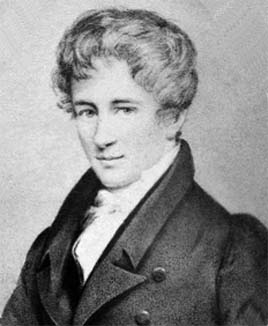# Abel's Impossibility Theorem

Spring 2019 - University of Illinois at ChicagoThe project is on Arnold’s proof of Abel’s theorem in which he provided a geometric explanation of the theorem. The proof demonstrates that we cannot construct a closed formula that produces the roots of general fifth degree polynomial using a finite combination of field operations, radicals, and elementary functions. Our goal is to showcase the most important aspects of Arnold’s proof. Using javascript we developed an animated webpage application that allows users to visually understand the main argument of Arnold’s proof. Specifically, it shows that given any expression f : {a0,...,a4} → C5 that uses elementary functions and radicals, one can construct a closed path in the space Poly5 (C) of monic fifth degree polynomials, such that all values of f return to their original positions, while the roots z1,...,z5 undergo a non-trivial permutation; therefore such f cannot reconstruct the roots z1,...,z5 from the coefficients a0,...,a4.